Learning Objectives

By the end of this section, you should be able to:

Select the rate-determining step given the consecutive reactions and overall rate law of the reaction

Derive the rate law of a reaction given the consecutive reactions

Derive the rate law under pre-equilibria conditions

$A\xrightarrow{\text{ka}}I\xrightarrow{\text{kb}}P$

This approximation assumes intermediate, I, is in a low constant concentration,ie:

$\frac{d[I]}{dt}=0$

Generally the approximation is more accurate when $k_{b}>>k_{a}$ ($k_{b}$ is much greater than $k_{a}$). The bigger the difference, the more accurate the assumption.

$k_{b}$ much greater than $k_{a}$ means that the reaction converts intermediate to product much faster than converting reactant to intermediate, then all the intermediate molecules will quickly be consumed after being produced. The intermediate molecule will stay at a relatively low concentration.

As we saw before with first order reactions: $\frac{d[A]}{dt}=-k_{a}[A]$ leads to $[A]=[A]_{0}e^{-k_{a}t}$

Now: $\frac{d[I]}{dt}=k_{a}[A]-k_{b}[I]≈0$ so $[I]=\frac{k_{a}}{k_{b}}[A]$

For product: $\frac{d[P]}{dt}=k_{b}[I]≈k_{a}[A]$ if we solved this, we would find: $[P]=[A]_{0}(1-e^{-k_{a}t})$

Detailed proof: This is not required for the course, but might be useful for understanding.

For reactant:

$\frac{d[A]}{dt}=-k_{a}[A]$

$[A]$ is a function of time. A function’s derivative is a multiple of itself, the function would be in an exponential form (will learn more of this in MATH 256 next term)

$[A]=constant*e^{-k_{a}t}$, to find the constant, we know that [A] is equal to $[A]_{0}$ at time 0. Substitute t=0; the exponential term is equal to 1. We are left with $[A]_{0}=constant$. Therefore:

$[A]=[A]_{0}*e^{-k_{a}t}$

For intermediate:

$\frac{d[I]}{dt}=k_{a}[A]-k_{b}[I]≈0$ so $[I]=\frac{k_{a}}{k_{b}}[A]$ (use the second equivalence of the equation, no calculus needed)

For product:

$\frac{d[P]}{dt}=k_{b}[I]≈k_{a}[A]$

\begin{align*}
\frac{d[P]}{dt} & = k_{a}[A] \\
\frac{d[P]}{dt} & = k_{a}*[A]_{0}*e^{-k_{a}t}\\
[P] & = \int k_{a}*[A]_{0}*e^{-k_{a}t}\\
[P] & = -[A]_{0}*e^{-k_{a}t} + constant
\end{align*}

To find the constant, we know that there is no product in the system, initially. At t=0, [P]=0. Subsitute t=0, the exponential term is equal to 1, so we are left with $0=-[A]_{0}+constant$, so $constant=[A]_{0}$, Therefore:

$[P]= -[A]_{0}*e^{-k_{a}t} + [A]_{0}=[A]_{0}(1-e^{-k_{a}t})$

Taking arbitrary values of $k_{a}=1$ and $k_{b}=10$, we can plot the concentration vs. time for all the components: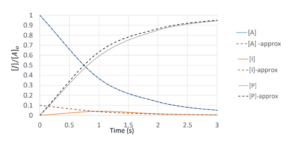Exercise: Using Steady-state Approximation to Find Rate Law Expressions

To derive the overall rate law for this reaction:

$2N_{2}O_{5(g)}→4NO_{2(g)}+O_{2(g)}$

Assume the reaction follows the following three-step mechanism: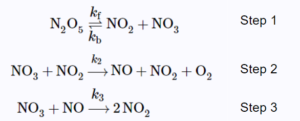The final expression of the rate law should only contain $[N_{2}O_{5}]$ and the rate constants of the elementary steps.$^{}$

### Solution

Step 1: Find the intermediates and express their rate of formation. Equate their rate of formation to 0 due to steady-state approximation.

$\frac{d[NO]}{dt}=k_{2}[NO_{3}][NO_{2}]-k_{3}[NO_{3}][NO]=0\;\;\;\;\;(1)$

$\frac{d[NO_{3}]}{dt}=k_{f}[N_{2}O_{5}]-k_{b}[NO_{2}][NO_{3}]-k_{2}[NO_{3}][NO_{2}]-k_{3}[NO_{3}][NO]=0\;\;\;\;\;(2)$

Step 2: Express the overall rate law. The easiest way is to express $\frac{d[O_{2}]}{dt}$ because it is a product that has stoichiometric coefficient of 1.

$\frac{d[O_{2}]}{dt}=k_{2}[NO_{3}][NO_{2}]$

Step 3: From the expression of $\frac{d[O_{2}]}{dt}$, we can see that to find the overall rate law, we need to find $[NO_{3}][NO_{2}]$ as an expression of the concentrations of reactants or products (no intermediates) by manipulating (1) and (2).

From (1):

$k_{2}[NO_{3}][NO_{2}]=k_{3}[NO_{3}][NO]$

Substitute into (2):
\begin{align*}
k_{f}[N_{2}O_{5}]-k_{b}[NO_{2}][NO_{3}]-k_{2}[NO_{3}][NO_{2}]-k_{2}[NO_{3}][NO_{2}] & = 0\\
k_{b}[NO_{2}][NO_{3}]+2k_{2}[NO_{3}][NO_{2}] & = k_{f}[N_{2}O_{5}]\\
[NO_{3}][NO_{2}] & =\frac{k_{f}[N_{2}O_{5}]}{k_{b}+2k_{2}}
\end{align*}

Step 4: We can substitute the expression for $[NO_{3}][NO_{2}]$ we just found into the expression for $\frac{d[O_{2}]}{dt}$, which is the same as the overall rate law.

$r=\frac{d[O_{2}]}{dt}=\frac{k_{2}k_{f}[N_{2}O_{5}]}{k_{b}+2k_{2}}$

## Rate-Determining Step

Rate determining step is the step that determines the overall rate of reaction in a series of reactions. The slowest forward reaction step is referred to as the rate-determining step, as it limits the rate of the entire reaction. Note that this must be a forward reaction step, a reverse reaction in an equilibrium reaction cannot be the rate-determining step.

An analogy that illustrates this concept is an hourglass having two different sized openings. The rate of the sand falling to the bottom-most chamber is determined by the smaller of the two openings. Similarly, the rate law of the overall reaction is determined from its rate-determining slowest step.$^{}$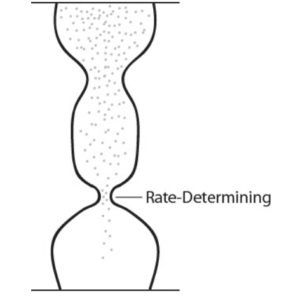## Pre-Equilibria

Say we have the following forward and reverse reaction: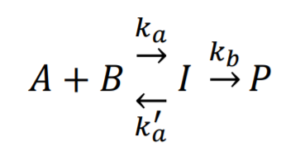Consider a case where an equilibrium is established due to $k_{a}’ >> k_{b}$:

The equilibrium constant, expressed using the forward and reverse reaction constants ($k_{a}$ and $k_{a}’$) would remain the same over time

The reaction of $I→P$ would decrease $[I]$ over time. Due to Le Châtelier’s principle, the equilibrium reaction will shift to produce more $I$

Because $k_{a}'>>k_{b}$, the reaction will shift concentrations to achieve equilibrium again far faster than the forward reaction ($k_{b}$) depletes $I$

Therefore, we can approximate $[I]$ with only the equilibrium part of the reaction

 $K=\Big(\frac{[I]}{[A][B]}\Big)_{eq}c^\theta=\frac{k_{a}}{k'_{a}}c^\theta$

Review: From lecture 4: reaction equilibrium:

The forward reaction: $A+B\xrightarrow{\text{ka}}I$, reaction rate $r=k_{a}[A][B]$

The reverse reaction: $I\xrightarrow{\text{k'a}}A+B$, reaction rate $r'=k_{a}'[I]$

At equilibrium: \begin{align*}
r & =r’\\
k_{a}[A][B] & = k_{a}'[I]\\
\frac{k_{a}}{k_{a}’}& = \frac{[I]}{[A][B]}
\end{align*}

So

 $[I]=\frac{K}{c^\theta}[A][B]=\frac{k_{a}}{k_{a}'}[A][B]$

Using the equation for $[I]$ based on this equilibrium, we can develop an equation for the rate of formation of $P$:

 $\frac{d[P]}{dt}=k_{b}[I]=k_{b}\frac{k_{a}}{k_{a}'}[A][B]=k_{r}[A][B]$

Where $k_{r}=k_{b}\frac{k_{a}}{k_{a}'}$. The final step of $I→P$ is rate determining, whereas the steps prior control the amount of intermediate.

### Relate $k_{r}$ to Activation Energy

Recall from Lecture 4:

For each step of the reaction, we can use the Arrhenius equation to substitute $k_{r}=Ae^{(-\frac{E_{a}}{RT})}$, where $A$ is the frequency factor and $E_{a}$ is the activation energy

So we can express the overall rate constant of the reaction above as:

$k_{r}=\frac{A_{a}e^{-\frac{Ea,a}{RT}}A_{b}e^{-\frac{Ea,b}{RT}}}{A_{a}'e^{-\frac{Ea,a'}{RT}}}=\frac{A_{a}A_{b}}{A_{a}'}e^{-\frac{(Ea,a+Ea,b-Ea,a')}{RT}}$

Although each rate constant may increase with temperature, this may not be true of the overall rate constant $k_{r}$.

The effective activation energy is: $E_{a}=E_{a}+E_{b}-E_{a}'$ and this may be positive or negative depending on $E_{a}$ of individual steps.

Exercise: Using Pre-Equilibria to Find the Rate Law Expression

The two-step mechanism below has been proposed for a reaction between nitrogen monoxide and molecular chlorine: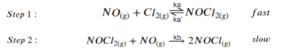Use this mechanism to derive the equation and predicted rate law for the overall reaction.

The rate law should be expressed in terms of $[NO]$, $[Cl_{2}]$, and the rate constants of the elementary reactions shown above.$^{}$

### Solution

Step 1: The overall reaction is the sum of the 2 elementary reactions:

$2NO_{(g)}+Cl_{2(g)}→2NOCl_{(g)}$

Step 2: Write each reaction separately and express the rate law for each reaction (including the reverse reaction in the equilibrium):

\begin{align*}
NO_{(g)}+Cl_{2(g)}→NOCl_{2(g)},\;\;\; & r_{1}=k_{a}[NO][Cl_{2}]\\
NOCl_{2(g)}→NO_{(g)}+Cl_{2(g)},\;\;\; & r_{-1}=k_{a}'[NOCl_{2}]\\
NOCl_{2}+NO→2NOCl,\;\;\; & r_{2}=k_{b}[NOCl_{2}][NO]
\end{align*}

Step 3: Use the equilibrium reaction to express the concentration of the intermediate ($[NOCl_{2}]$):

$[NOCl_{2}]=\frac{k_{a}}{k_{a}'}[NO][Cl_{2}]$

Step 4: Express the overall reaction rate using the step 2 elementary reaction, then subsitute in $[NOCl_{2}]$:
\begin{align*}
overall\;rate&=\frac{1}{2}\frac{d[NOCl]}{dt}\\
&=\frac{2k_{b}[NO][NOCl_{2}]}{2}\\
&=k_{b}[NO][NOCl_{2}]\\
&=\frac{k_{b}k_{a}}{k_{a}’}[NO]^2[Cl_{2}]
\end{align*}

## References

 Chemistry LibreTexts. 2020. Steady State Approximation. [online] Available at: <https://chem.libretexts.org/Bookshelves/Physical_and_Theoretical_Chemistry_Textbook_Maps/Supplemental_Modules_(Physical_and_Theoretical_Chemistry)/Kinetics/Reaction_Mechanisms/Steady-State_Approximation> [Accessed 04 May, 2020].

 Introduction to Chemistry. 1st Canadian Edition. 2014. Reaction Mechanisms. [online] Available at: <https://opentextbc.ca/introductorychemistry/chapter/reaction-mechanisms-2/> [Accessed 04 May, 2020].

 OpenStax Chemistry 2e. 2019. 12.6 Reaction Mechanisms. [online] Available at: <https://openstax.org/books/chemistry-2e/pages/12-6-reaction-mechanisms> [Accessed 05 May, 2020].